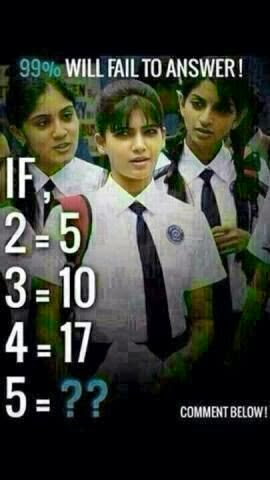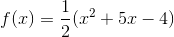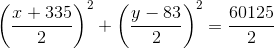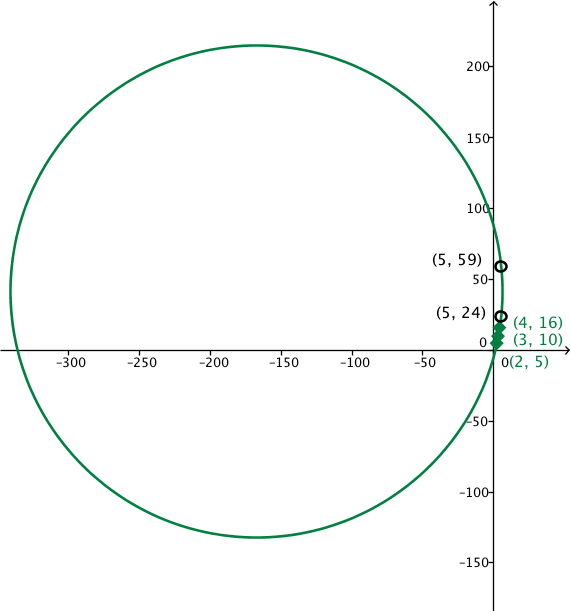# Are we that bad at math?

I get a little upset when I see some of these social media posts that challenge people to do simple math. This one was particularly bugging me.First, let's start with the obvious errors, 2 is not equal to 5, 3 is not equal to 10, and 4 is not equal to 16. If those things were true, then we know that 5=2 by the reflexive property.
I don't believe that is the answer that is expected, and I don't like redefining a basic piece of notation.
So l we need to fix this problem a little. I going to assume that they are trying to represent some relation by using the equals sign. I'm going to rewrite this:
 x y 2 5 3 10 4 17 5 ??
So looking for any type of patterns, I notice right away it isn't linear. The differences between the outputs are 5 and 7, so maybe the answer could be 26, by adding 9, given the little information that is given.
y = 26
However, there are many functions that can fit just three points.
Here is a parabola,that fits these points. Using this function f(5) = 23.And then there is this circle:. There are two answers here: 24 or 59.So so far we have answers of 2, 23, 24, 26, and 59. Are there more? Probably. I bet some sort of periodic function could fit those three points. Comment if you think you know of another answer.